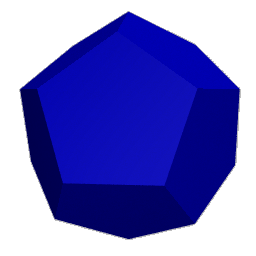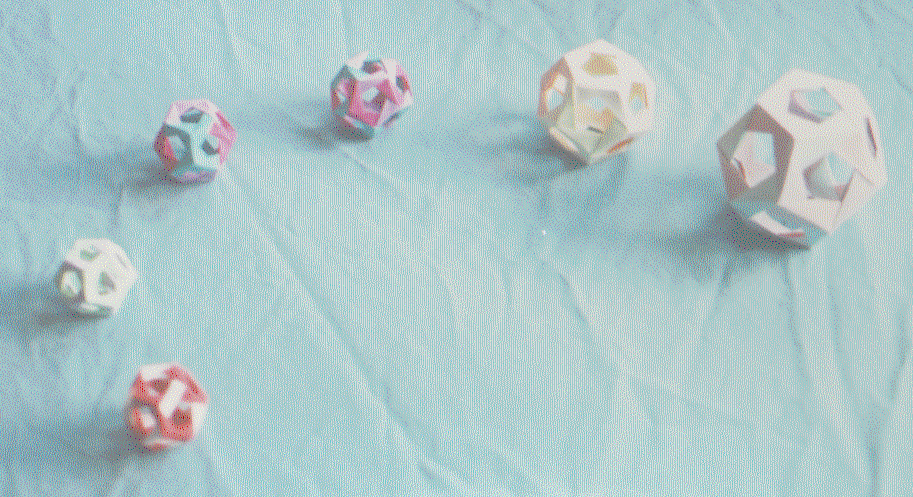# Making a dodecahedron

Introduction -- Making the Modules -- Making the Polyhedra -- Jim's Origami Page
The dodecahedron has 12 pentagonal faces that meet in groups of three at each of 20 vertices. It has 30 edges, and thus uses 30 identical pentagon (pe--pe) modules.

This is a great piece of origami -- simple to make, and rock solid. It is a good one upon which to learn how to use these modules. There are a couple of neat colorations here. They mostly use ten modules each of three different colors. One way is color such that no two adjacent edges on a pentagonal face are the same. The one I like better is to make the top and bottom pentagons out of color 1. Then have the 10 edges emanating from the top and bottom pentagons be color 2. The remaining ten edges of color 3 form a band around the middle. You can use this same design with two colors by making the band around the middle from color 1. Finally, you can color again with ten modules each of three different colors in the following way: Take five modules of one color, and fit them together as follows:

```                 \   /
---
/   \
```
Do that with the remaining pieces so that you have 6 composites like the one above, two of each color. These will fit together to make a dodecahedron with each pair of composites on opposite ends of the dodecahedron. (This is related to inscribing a cube in a dodecahedron).

## The dodecahedron## Six Penultimate DodecahedraJim Plank --- Jim's Origami Page